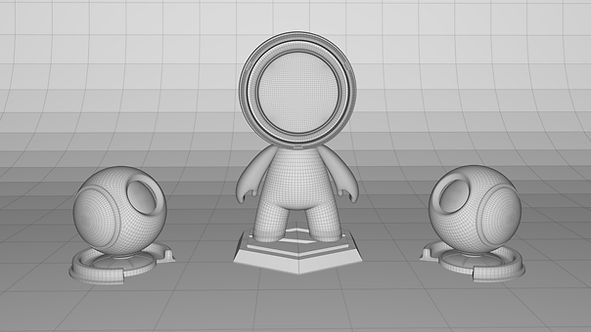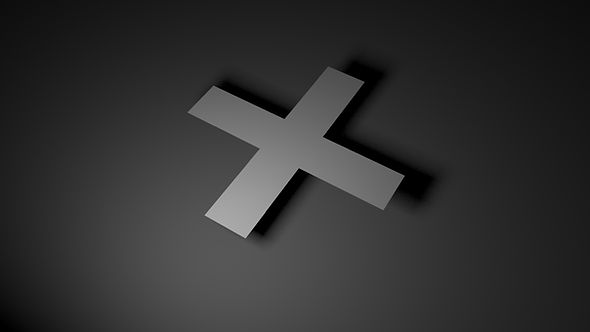top of pageThese images show the results of using the Open Shading Language (OSL) to write a variety of shaders for use with RenderMan for Maya.

My initial concept was to create very stylized and vibrant shaders, largely inspired by the work of Yayoi Kusama. The MAT model is taken from here.

Later I implemented the same logic and patterns with a little permutations to create flamboyant and experimental designs for a necktie.

# RendersOcclusion RenderVisualizer Render

Occlusion Render

Visualizer Render// A barebones example showing how to access 'st' texture

// coordinates and how to output a color. The shader assumes

// the values of 's' and 't' are in the range 0 to 1.

Cross(

float s = 0

[[

int lockgeom = 0,

string widget = "null",

]],

float t = 0

[[

int lockgeom = 0,

string widget = "null",

]],

float s_width = 0.2,

float s_mid = 0.5,

float t_width = 0.2,

float t_mid = 0.5,

color pat_color = color(1,0,0),

color bak_color = color(1,1,0),

output color resultRGB = 0)

{

float t_max = t_mid + t_width/2;

float t_min = t_mid - t_width/2;

float s_max = t_mid + t_width/2;

float s_min = t_mid - t_width/2;

if(t >= t_min && t<= t_max || s >= s_min && s <= s_max)

resultRGB = pat_color;

else

resultRGB = bak_color;

}// A barebones example showing how to access 'st' texture

// coordinates and how to output a color. The shader assumes

// the values of 's' and 't' are in the range 0 to 1.

float s = 0

[[

int lockgeom = 0,

string widget = "null",

]],

float t = 0

[[

int lockgeom = 0,

string widget = "null",

]],

float s_position = 0.5,

float t_position = 0.5,

float r_max = 0.75,

float r_min = 0.35,

color pat_color = color(1, 1, 1),

color bak_color = color(0, 1, 0),

output color resultRGB = 0)

{

float ss = s - floor(s);

float tt = t - floor(t);

float dist = distance(point(s_position,t_position,0), point(ss,tt,0));

if(dist <= r_max && dist >= r_min)

resultRGB = pat_color;

else

resultRGB = bak_color;

}// A barebones example showing how to access 'st' texture

// coordinates and how to output a color. The shader assumes

// the values of 's' and 't' are in the range 0 to 1.

float s = 0

[[

int lockgeom = 0,

string widget = "null",

]],

float t = 0

[[

int lockgeom = 0,

string widget = "null",

]],

float s_position = 0.5,

float t_position = 0.5,

float r_max = 0.5,

float r_min = 0.4,

color pat_color = color(1, 1, 1),

color bak_color = color(0, 1, 0),

output color resultRGB = 0)

{

float ss = s - floor(s);

float tt = t - floor(t);

float dist = distance(point(s_position,t_position,0), point(ss,tt,0));

if(dist <= r_max && dist >= r_min)

resultRGB = pat_color;

else

resultRGB = bak_color;

}

# Used in a Shader Networki. PxrManifold2D : I used PxrManifold2D to multiply the S and T values to scale the repetitions.

ii. PxrFractal : I used the PxrFractal node to slightly offset the positions, via the attribute called stagger.

iii. PxrOSL : The PxrOSL node imports the OSL code. I connected the ResultRGB of the PxrOSL to Pat Color attribute (Pattern Color) to layer up the effects of multiple OSL shaders. The same shaders can also be used as a mask.

iv. UV : For the OSL to work seamlessly, the object should be properly UVed.

v.  PxrNoise : PxrFractal or Voronoi noises can be plugged in into attributes like radius to get an randomization of the radius or the respective attribute.

The same can be done through MEL scripting.

# Final Thoughts

This was the first time I used OSL to create shading networks. The amount of available control that OSL gives is quite vast, as it can influence various attributes. In my experience this led a lot of experimentation and happy accidents. It would have been ideal if the OSL output could  be directly visualized in the viewport itself rather than rendering it every time to check the results.

If I have had more time I would use it direct towards the following:

i. Implementing displacement.

ii. Apply animation for shaders and Pxr noises.

iii. Have more minute pattern variations (as seen in various fabrics or carpet designs).

iv. Creating a whole set of shaders for formal clothing (blazer, formal shirt and pants with the above tie) for an even better context.

v.  Create more experimental and interesting patterns.